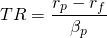# Treynor ratio

Measuring the performance of a portfolio over time by just looking at its profitability is not a good idea. The reason is that different portfolios can have obtained other profits even though they all have the same underlying strategy. A good performance metric thus incorporates the risk component. The Treynor ratio is an often used risk-adjusted performance. Similarly to the Sharpe ratio, it is also a the return-to-variability ratio. However in this case, adjusted for systematic risk. This metric thus indicates the amount of return a portfolio is earning in excess of the risk free rate adjusted for systematic risk. Specifically, this means that portfolios with a higher the portfolio return have a higher Treynor ratio for a certain risk free rate systematic risk component. On the other hand, the lower the systematic risk of the portfolio the better its the performance.

## Treynor ratio formula

The calculation of the Treynor ratio is somewhat similar is the Sharpe ratio. It can be calculated by subtracting the risk free rate from the portfolio return. This result is then further adjusted for the taken systematic risk indicated by the β.## Treynor ratio and risk free rate

The risk free rate stated in the Treynor ratio is a theoretical concept and doesn’t exist in reality. However, in practice often the 3-month T-Bill or the Libor rate is used as the risk free rate. Retail investors could also opt for using the interest rate of their savings account. Implying that this is their best ‘risk-free’ alternative. Any investment should thus in the long-run beat the return earned on a regular savings account.

## Treynor ratio problems

As shown by the formula above, the Treynor ratio penalizes a portfolios performance by its exposure to systematic risk. Therefore, non-well diversified portfolios will seem to have a good performance. However, it should be kept in mind that these portfolios actually bear more risk than its systematic part shows. Moreover, this hidden risk is not compensated by higher returns.

## Summary

The Treynor ratio is a risk-adjusted portfolio performance metric. It indicates the extra return a portfolio is earning for taking an additional unit in systematic risk.